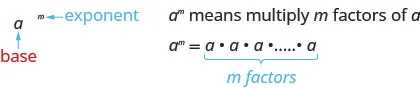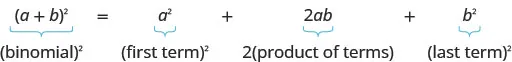Elementary Algebra 2e

# Key Concepts

### Key Concepts

• Monomials
• A monomial is a term of the form $axmaxm$, where $aa$ is a constant and $mm$ is a whole number
• Polynomials
• polynomial—A monomial, or two or more monomials combined by addition or subtraction is a polynomial.
• monomial—A polynomial with exactly one term is called a monomial.
• binomial—A polynomial with exactly two terms is called a binomial.
• trinomial—A polynomial with exactly three terms is called a trinomial.
• Degree of a Polynomial
• The degree of a term is the sum of the exponents of its variables.
• The degree of a constant is 0.
• The degree of a polynomial is the highest degree of all its terms.

#### 6.2Use Multiplication Properties of Exponents

• Exponential Notation• Properties of Exponents
• If $a,ba,b$ are real numbers and $m,nm,n$ are whole numbers, then
$Product Propertyam·an=am+nPower Property(am)n=am·nProduct to a Power(ab)m=ambmProduct Propertyam·an=am+nPower Property(am)n=am·nProduct to a Power(ab)m=ambm$

#### 6.3Multiply Polynomials

• FOIL Method for Multiplying Two Binomials—To multiply two binomials:
1. Step 1. Multiply the First terms.
2. Step 2. Multiply the Outer terms.
3. Step 3. Multiply the Inner terms.
4. Step 4. Multiply the Last terms.

• Multiplying Two Binomials—To multiply binomials, use the:
• Multiplying a Trinomial by a Binomial—To multiply a trinomial by a binomial, use the:

#### 6.4Special Products

• Binomial Squares Pattern
• If $a,ba,b$ are real numbers,• $(a+b)2=a2+2ab+b2(a+b)2=a2+2ab+b2$
• $(a−b)2=a2−2ab+b2(a−b)2=a2−2ab+b2$
• To square a binomial: square the first term, square the last term, double their product.

• Product of Conjugates Pattern
• If $a,ba,b$ are real numbers,• $(a−b)(a+b)=a2−b2(a−b)(a+b)=a2−b2$
• The product is called a difference of squares.

• To multiply conjugates:
• square the first term square the last term write it as a difference of squares

#### 6.5Divide Monomials

• Quotient Property for Exponents:
• If $aa$ is a real number, $a≠0a≠0$, and $m,nm,n$ are whole numbers, then:
$aman=am−n,m>nandaman=1am−n,n>maman=am−n,m>nandaman=1am−n,n>m$
• Zero Exponent
• If $aa$ is a non-zero number, then $a0=1a0=1$.

• Quotient to a Power Property for Exponents:
• If $aa$ and $bb$ are real numbers, $b≠0,b≠0,$ and $mm$ is a counting number, then:
$(ab)m=ambm(ab)m=ambm$
• To raise a fraction to a power, raise the numerator and denominator to that power.

• Summary of Exponent Properties
• If $a,ba,b$ are real numbers and $m,nm,n$ are whole numbers, then
$Product Propertyam·an=am+nPower Property(am)n=am·nProduct to a Power(ab)m=ambmQuotient Propertyambm=am−n,a≠0,m>naman=1an−m,a≠0,n>mZero Exponent Definitionao=1,a≠0Quotient to a Power Property(ab)m=ambm,b≠0Product Propertyam·an=am+nPower Property(am)n=am·nProduct to a Power(ab)m=ambmQuotient Propertyambm=am−n,a≠0,m>naman=1an−m,a≠0,n>mZero Exponent Definitionao=1,a≠0Quotient to a Power Property(ab)m=ambm,b≠0$

#### 6.6Divide Polynomials

• If $a,b,andca,b,andc$ are numbers where $c≠0c≠0$, then
$ac+bc=a+bcanda+bc=ac+bcac+bc=a+bcanda+bc=ac+bc$

• Division of a Polynomial by a Monomial
• To divide a polynomial by a monomial, divide each term of the polynomial by the monomial.

#### 6.7Integer Exponents and Scientific Notation

• Property of Negative Exponents
• If $nn$ is a positive integer and $a≠0a≠0$, then $1a−n=an1a−n=an$
• Quotient to a Negative Exponent
• If $a,ba,b$ are real numbers, $b≠0b≠0$ and $nn$ is an integer , then $(ab)−n=(ba)n(ab)−n=(ba)n$
• To convert a decimal to scientific notation:
1. Step 1. Move the decimal point so that the first factor is greater than or equal to 1 but less than 10.
2. Step 2. Count the number of decimal places, $nn$, that the decimal point was moved.
3. Step 3.
Write the number as a product with a power of 10. If the original number is:
• greater than 1, the power of 10 will be $10n10n$
• between 0 and 1, the power of 10 will be $10−n10−n$
4. Step 4. Check.

• To convert scientific notation to decimal form:
1. Step 1. Determine the exponent, $nn$, on the factor 10.
2. Step 2.
Move the decimal $nn$places, adding zeros if needed.
• If the exponent is positive, move the decimal point $nn$ places to the right.
• If the exponent is negative, move the decimal point $|n||n|$ places to the left.
3. Step 3. Check.
Order a print copy

As an Amazon Associate we earn from qualifying purchases.